# Best line to fit three points

Suppose you want to fit a line to three data points. If no line passes exactly through your points, what’s the best compromise you could make?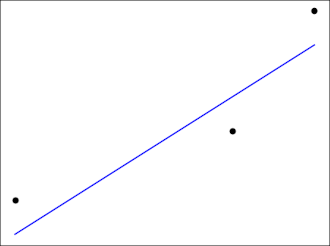Chebyshev suggested the best thing to do is find the minmax line, the line that minimizes the maximum error. That is, for each candidate line, find the vertical distance from each point to the line, and take the largest of these three distances as the measure of how well the line fits. The best line is the that minimizes this measure.

Note that this is not the same line you would get if you did a linear regression. Customary linear regression minimizes the average squared vertical distance from each point to the line. There are reasons this is the standard approach when you have at least a moderate amount of data, but when you only have three data points, it makes sense to use the minmax approach.

We can say several things about the minmax line. For one thing, there exists such a line and it is unique. Also, the vertical distance from each data point to the line will be the same. Either two points will be over the line and one under, or two will be under and one over.

Suppose your three points are (x1, y1), (x2, y2), and (x3, y3), with x1 < x2 < x3. Then the slope will beand the intercept will beI made an online calculator to find the best line for three points.

# How well does a spline fit a function?

Suppose you’re going to fit a spline s to a function f by interpolating f at a number of points. What can you know a priori about how well s will approximate f?

This question was thoroughly resolved five decades ago , but the result is a bit complicated, so we’ll incrementally work our way up to the final result.

## What kind of spline

First of all we’ll need to be specific about what we mean by a spline. We’re going to look at cubic splines over a finite interval [a, b]. A cubic spline approximation to f over [a, b] is constructed by splitting the interval into N subintervals and fitting a cubic polynomial to f over each subinterval. The piecewise polynomials much match the value of f at the ends of the subintervals, and the values over contiguous intervals much match their first and second derivatives.

We’re not going to specify the endpoint conditions of the spline. The spline described above is not unique; it has two degrees of freedom which are typically specified by boundary conditions, but the theorem we’re building up to doesn’t require us to say anything about boundary conditions. In particular, we do not require our spline to be a natural cubic spline.

## How to measure fit

How should we measure how well our spline s fits our function f? Obviously we’d like have a bound on

|s(x) – f(x)|

at each point. We’d also like to have a bound on the difference in derivatives at each point. Since our spline has two continuous derivatives, we’re interested in the first and second derivatives. In symbols, we’d like to bound

|s(r)(x) – f(r)(x)|

for r = 0, 1, or 2.

## Smoothness of f

We have to say something about the smoothness of the function we’re interpolating. Since we’re appoximating f by a function with two continuous derivatives, it only makes sense to consider functions f with at least two derivatives. Typically f will be smoother than s, so we will also look at the case of f having three or four continuous derivatives. We will measure the smoothness of f by the maximum value of its derivatives over the interval [a, b]. That is, by

|f (m)|

where m = 2, 3, or 4.

## Mesh size

Obviously the accuracy of our approximation will depend on the number of interpolation points N+1. We will not require the interpolation points to be evenly spaced, and so we will quantify the fineness of our mesh as the length h of the longest subinterval of [a, b].

## Shape of our theorem

We now have the elements we need to state our theorem. The left side will be

|s(r)(x) – f(r)(x)|

and the right side will be some expression involving r, n, h, and the values of

|f (m)|

for varying values of m.

We can make the bound on the left side tighter by taking into account which subinterval j = 0, 1, 2, … N-1 our x belongs to. In general, spline interpolation is more accurate toward the middle of [a, b] than toward the edges. Interestingly, our theorem will only depend on j and not on the width of the jth interval.

## The theorem

And now for the theorem we’ve been working our way up to:

|s(r)(x) – f(r)(x)| ≤ εmr |f(m)|hmr+ Kmβr (21-j + 21-N+j) h.

The statement of the theorem is complicated because the author is carefully quantifying the error. You could make the theorem much simpler by just making a big-O statement. Instead, the author gives numerical values of each of the constants.

The theorem is even a little more complicated than it looks at first because the constants Km depend on |f(m)|h. The values of the constants in the theorem are given in the table below. You can click on the image to get a larger image that is easier to read.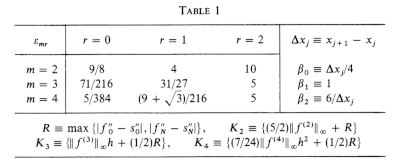## More spline posts

 C. A. Hall. Natural Cubic and Bicubic Spline Interpolation. SIAM Journal on Numerical Analysis , Dec., 1973, Vol. 10, No. 6 (Dec., 1973), pp. 1055–1060

# Error estimates for splines with more boundary conditions

Yesterday I wrote about rates of convergence for natural cubic splines. This brief post reports similar results for more boundary conditions.

As explained in the earlier post, a cubic spline that interpolates a function f at n+1 points satisfies 4n -2 equations in 4n variables. Two more equations are necessary to uniquely determine the interpolating cubic spline.

A natural cubic spline S over [a, b] satisfies

S”(a) = 0
S”(b) = 0.

There are other ways of coming up with two more equations. You might match first derivatives at the end points

S‘(a) = f‘(a)
S‘(b) = f‘(b)

or second derivatives

S”(a) = f”(a)
S”(b) = f”(b).

Or if f is periodic you could require first and second derivatives of the spline to match at a and b.

S‘(a) = S‘(b)
S”(a) = S”(b).

In each case, you get the same order of convergence as we reported earlier for the natural cubic spline, assuming f is 4 times continuously differentiable over [a, b]. The error goes down like O(1/n4).

Source: D. Kershaw. A Note on the Convergence of Interpolatory Cubic Splines. SIAM Journal on Numerical Analysis, Mar., 1971, Vol. 8, No. 1 pp. 67-74.

## Example

When we use natural cubic splines to fit exp(x) over [0, 2π] in the earlier post, the error was fairly large. We get better results when we specify the derivatives at the two ends of the interval. Here’s what we get for 11 points: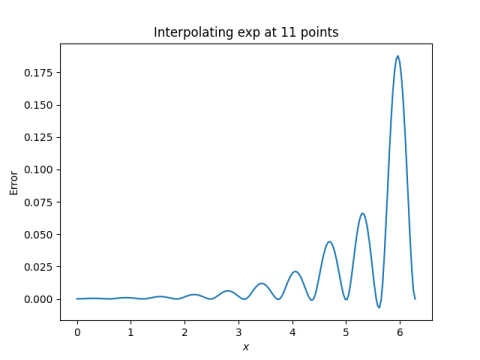The error is about 7 times smaller than with natural cubic splines.

And here’s what we get for 21 points: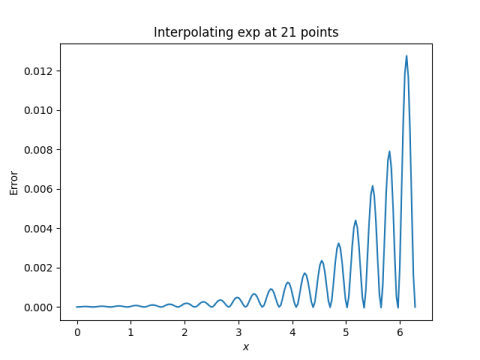In this case the error is about 8x smaller than with natural cubic splines.

When we go from 11 to 21 points, specifying the derivatives on each end, the error drops by about a factor of 15, close value of 16 we’d expect in the limit.

Incidentally, to specify the boundary conditions of the spline in Python we need to add an option parameter bc_type in the call to CubicSpline. Otherwise the code is the same as before. In our example

    bc_type=((1, 1), (1, np.exp(2*np.pi)))

We set bc_type equal to a pair of pairs. The first pair gives a boundary condition on the left end and the second gives a boundary condition on the right. The first argument of the inner pair in both cases is 1, because we’re specifying 1st derivatives. Use a 2 to specify 2nd derivatives. The second argument to the pair the value of the derivative: exp(0) = 1 on the left, and exp(2π) on the right.

# Rate of natural cubic spline convergence

Suppose you want to approximate a function with a polynomial by interpolating it at evenly spaced points. You might reasonably expect that the more points you use, the better the approximation will be. That might be true, but it might not. As explained here, for some functions the maximum approximation error actually increases as the number of points increases. This is called the Runge phenomenon.

What about cubic splines? Instead of fitting one high-degree polynomial, you fit a different cubic polynomial on each sub-interval, with the constraint that the pieces fit together smoothly. Specifically, the spline must be twice differentiable. This means that the first and second derivatives from both sides match at the end of each interval.

Say our function f is defined over an interval [a, b] and we break the interval into n sub-intervals. This means we interpolate f at n+1 evenly spaced points. We have 4n degrees of freedom: four polynomial coefficients over each interval. Interpolation adds 2n constraints: the value of the polynomial is specified at the ends of each sub-interval. Matching first and second derivatives at each of the n-1 interior nodes gives 2(n-1) constrains. This is a total of 4n – 2 equations for 4n unknowns.

We get the two more equations we need by specifying something about the derivatives at a and b. A natural cubic spline sets the second derivatives equal to zero at a and b.

So if we interpolate f at n+1 evenly spaced points using a natural cubic spline, does the splines converge uniformly to f as we increase n? Indeed they do. Nothing like Runge phenomenon can happen. If f is four times continuously differentiable over [ab], then convergence is uniform on every compact subinterval of (ab) and the rate of convergence is O(1/n4). If the second derivative of f is zero at a and b, then the rate of convergence is O(1/n4) over the whole interval [a, b].

Source: Kendall E. Atkinson. On the Order of Convergence of Natural Cubic Spline Interpolation. SIAM Journal on Numerical Analysis, Vol. 5, No. 1 (Mar., 1968), pp. 89-101.

Update: See this post for results for other kinds of cubic splines, i.e. cubic splines with different boundary conditions.

## Experiment

We will look at sin(x) and exp(x) on the interval [0, 2π]. Note that the second derivatives of former at zero at both ends of the interval, but this is not true for the latter.

We will look at the error when interpolating our functions at 11 points and 21 points (i.e. 10 sub-intervals and 20 sub-intervals) with natural cubic splines. For the sine function we expect the error to go down by about a factor of 16 everywhere. For the exponential function, we expect the error to go down by about a factor of 16 in the interior of [0, 2π]. We’ll see what happens when we zoom in on the interval [2, 4].

We’re basing our expectations on a theorem about what happens as the number of nodes n goes to infinity, and yet we’re using fairly small values of n. So we shouldn’t expect too close of an agreement with theory, but we’ll see how close our prediction gets.

## Computation

The following Python code will plot the error in the interpolation by natural cubic splines.

    import numpy as np
from scipy.interpolate import CubicSpline
import matplotlib.pyplot as plt

for n in [11, 21]:
for f in [np.sin, np.exp]:
knots = np.linspace(0, 2*np.pi, n)
cs = CubicSpline(knots, f(knots))
x = np.linspace(0, 2*np.pi, 200)

plt.plot(x, f(x) - cs(x))
plt.xlabel("$x$")
plt.ylabel("Error")
plt.title(f"Interpolating {f.__name__} at {n} points")
plt.savefig(f"{f.__name__}_spline_error_{n}.png")
plt.close()


Notice an interesting feature of NumPy: we call the __name__ method on sin and exp to get their names as strings to use in the plot title and in the graphic file.

Here’s what we get for the sine function.

First for 10 intervals: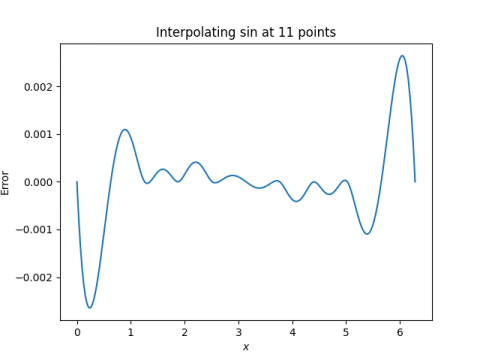Then for 20 intervals:When we doubled the number of intervals, the maximum error went down by about 30x, better than the theoretical upper bound on error.

Here’s what we get for the exponential function.

First for 10 intervals:Then for 20 intervals: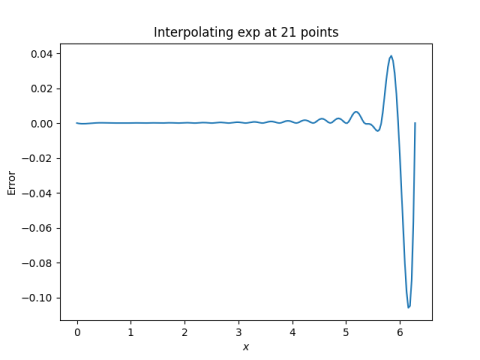The error in approximating the exponential function by splines is much greater than the error in approximating the sine function. That’s because the former acts less like a polynomial than the latter. Polynomial approximation, and piecewise polynomial approximation, works better on things that behave like polynomials.

When we doubled the number of intervals, the maximum error went down by a factor of 12. We shouldn’t be surprised that the error to go down by a factor of less than 16 since the hypotheses for the theorem that gives a factor of 16 aren’t satisfied.

Here’s what we get when we zoom in on the interval [2, 4].

For 10 subintervals: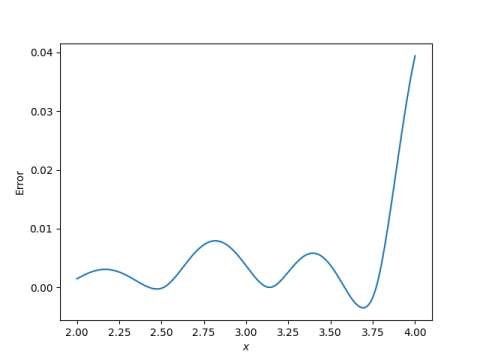And for 20 subintervals: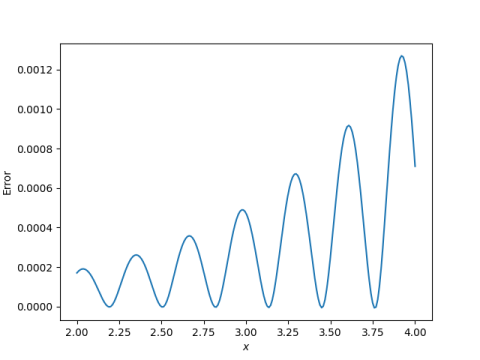The error is much smaller over the interior interval. And when we double the number of interpolation points, the error over [2, 4] goes down by about a factor of 3 just as it did for sine.

Note that in these last two plots, we’re still interpolating over the entire interval [0, 2π], but we’re looking at the error over [2, 4]. We’re zooming in on part of the error plot, not allocating our interpolation points specifically to the smaller interval.

# Monotonic interpolation

Accuracy isn’t everything. Sometimes when you’re approximating a function you care more about matching a function’s qualitative behavior than matching its numerical values.

One example is interpolating monotonic data. Say your data show that increasing input always increases output. It’s likely you want a function that interpolates your data to have the same property and that you might sacrifice some accuracy to assure monotonicity. In a visual application, you might get a distracting wiggle in what should be a smoothly bending curve. In software used to control a physical device, it could lead to unexpected behavior, or even disaster, if an increase in input led to a decrease in output, even if it was a small decrease.

Linear interpolation preserves monotonicity: if in your data increasing x leads to increasing y, the same is true of a linear interpolation to the data. But for higher-order interpolation this isn’t the case. A simple example would be the three points (-1, 1), (2, 4), and (3, 9). The unique quadratic polynomial interpolating these three points is x², which is not monotonic in any interval containing 0. We’d like to have a smoother and more accurate  way to interpolate data while still preserving monotonicity.

It’s unlikely that a polynomial interpolating monotone data will be monotone. I suspect that given a set of monotonic data, it’s more likely that a polynomial spline interpolant will be monotone than a polynomial interpolant because the former is more flexible. Formulating this suspicion into a conjecture that could be settled theoretically, or even tested empirically, would be a non-trivial but interesting exercise.

A cubic spline interpolating monotone data is not necessarily monotone. However, there is a way to determine derivative conditions so that a Hermite spline fitting the data will be monotone. Fritsch and Carleson published an algorithm for this in 1980.

## Related posts

 If the function sampled by your data is smooth, then in general a smooth approximation to the function will be more accurate than a piecewise linear approximation.

# Tridiagonal systems, determinants, and natural cubic splines

## Tridiagonal matrices

A tridiagonal matrix is a matrix that has nonzero entries only on the main diagonal and on the adjacent off-diagonals. This special structure comes up frequently in applications. For example, the finite difference numerical solution to the heat equation leads to a tridiagonal system. Another application, the one we’ll look at in detail here, is natural cubic splines. And we’ll mention an interesting result with Fibonacci numbers in passing.

Because these matrices have a special structure, the corresponding linear systems are quick and easy to solve. Also, we can find a simple recurrence relation for their determinants.

## Determinants

Let’s label the component of a tridiagonal matrix as belowwhere every component not shown is implicitly zero. We can expand the determinant of the matrix above using minors along the last row. This gives a recursive expression for the determinantwith initial conditions d0 = 1 and d-1 = 0.

Note that if all the a‘s and b‘s are 1 and all the c‘s are -1, then you get the recurrence relation that defines the Fibonacci numbers. That is, the Fibonacci numbers are given by the determinant## Natural cubic splines

A cubic spline interpolates a set of data points with piecewise cubic polynomials. There’s a (potentially) different cubic polynomial over each interval between input values, all fitted together so that the resulting function, its derivative, and its second derivative are all continuous.

Suppose you have input points, called knots in this context, t0, t1, … tn and output values y0, y1, … yn. For the spline to interpolate the data, its value at ti must be yi.

A cubic spline then is a set of n cubic polynomials, one for each interval [ti, ti+1]. A cubic polynomial has four coefficients, so we have 4n coefficients in total. At each interior knot, t1 through tn-1, we have four constraints. Both polynomials that meet at ti must take on the value yi at that point, and the two polynomials must have the same first and second derivative at that point. That gives us 4(n-1) equations. The value of the first polynomial is specified on the left end at t0 the value of the last polynomial is specified at the right end at tn. This gives us 4n – 2 equations in total.

We need two more equations. A clamped cubic spline specifies the derivatives at each end point. The natural cubic spline specifies instead that the second derivatives at each end are zero. What is natural about a natural cubic spline? In a certain sense it is the smoothest curve interpolating the specified points. With these boundary conditions we now have as many constraints as degrees of freedom.

So how would we go about finding the coefficients of each polynomial? Our task will be much easier if we parameterize the polynomials cleverly to start with. Instead of powers of x, we want powers of (xti) and (ti+1 – x) because these expressions are 0 on different ends of the interval [ti, ti+1]. It turns out we parameterize the spline over the ith interval aswhere hi = ti+1ti, the length of the ith interval.

This may seem unmotivated, and no doubt it is cleaner than the first thing someone probably tried, but it’s the kind of thing you’re lead to when you try to make the derivation work out smoothly.

The basic form is  powers of (xti) and (ti+1 – x), each to the first and third powers, for reasons given above. Why the 6’s in the denominators? They’re not strictly necessary, but they cancel out when we take second derivatives. Let’s look at the second derivative.Note how when we stick in ti the first term is zero and the second is zi, and when we stick in ti+1 the first term is zi+1 and the second is zero.

We can now write down the system of equations for the z‘s. We have z0 = zn from the natural cubic spline condition, and for 1 ≤ in-1 we havewhereNote that this is a tridiagonal system because the ith equation only involves z‘s with subscripts i-1, i, and i+1.

Because of its tridiagonal structure, these equations can be solved simply and efficiently, much more efficiently than a general system of equations.

# Runge phenomena

I’ve mentioned the Runge phenomenon in a couple posts before. Here I’m going to go into a little more detail.

First of all, the “Runge” here is Carl David Tolmé Runge, better known for the Runge-Kutta algorithm for numerically solving differential equations. His name rhymes with cowabunga, not with sponge.

Runge showed that polynomial interpolation at evenly-spaced points can fail spectacularly to converge. His example is the function f(x) = 1/(1 + x²) on the interval [-5, 5], or equivalently, and more convenient here, the function f(x) = 1/(1 + 25x²) on the interval [-1, 1]. Here’s an example with 16 interpolation nodes.Runge found that in order for interpolation at evenly spaced nodes in [-1, 1] to converge, the function being interpolated needs to be analytic inside a football-shaped  region of the complex plane with major axis [-1, 1] on the real axis and minor axis approximately [-0.5255, 0.5255]  on the imaginary axis. For more details, see .

The function in Runge’s example has a singularity at 0.2i, which is inside the football. Linear interpolation at evenly spaced points would converge for the function f(x) = 1/(1 + x²) since the singularity at i is outside the football.For another example, consider the function f(x) = exp(- 1/x²) , defined to be 0 at 0. This function is infinitely differentiable but it is not analytic at the origin. With only 16 interpolation points as above, there’s a small indication of trouble at the ends.With 28 interpolation points in the plot below, the lack of convergence is clear.The problem is not polynomial interpolation per se but polynomial interpolation at evenly-spaced nodes. Interpolation at Chebyshev points converges for the examples here. The location of singularities effects the rate of convergence but not whether the interpolants converge.

RelatedHelp with interpolation

***

 American football, that is. The region is like an ellipse but pointy at -1 and 1.

 Approximation Theory and Approximation Practice by Lloyd N. Trefethen

# Chebyshev interpolation

Fitting a polynomial to a function at more points might not produce a better approximation. This is Faber’s theorem, something I wrote about the other day.

If the function you’re interpolating is smooth, then interpolating at more points may or may not improve the fit of the interpolation, depending on where you put the points. The famous example of Runge shows that interpolating

f(x) = 1 / (1 + x²)

at more points can make the fit worse. When interpolating at 16 evenly spaced points, the behavior is wild at the ends of the interval.Here’s the Python code that produced the plot.

    import matplotlib.pyplot as plt
from scipy import interpolate, linspace

def cauchy(x):
return (1 + x**2)**-1

n = 16
x = linspace(-5, 5, n) # points to interpolate at

y = cauchy(x)
f = interpolate.BarycentricInterpolator(x, y)

xnew = linspace(-5, 5, 200) # points to plot at
ynew = f(xnew)
plt.plot(x, y, 'o', xnew, ynew, '-')
plt.show()


However, for smooth functions interpolating at more points does improve the fit if you interpolate at the roots of Chebyshev polynomials. As you interpolate at the roots of higher and higher degree Chebyshev polynomials, the interpolants converge to the function being interpolated. The plot below shows how interpolating at the roots of T16, the 16th Chebyshev polynomial, eliminates the bad behavior at the ends.To make this plot, we replaced x above with the roots of T16, rescaled from the interval [-1, 1] to the interval [-5, 5] to match the example above.

    x = [cos(pi*(2*k-1)/(2*n)) for k in range(1, n+1)]
x = 5*array(x)


What if the function we’re interpolating isn’t smooth? If the function has a step discontinuity, we can see Gibbs phenomena, similar to what we saw in the previous post. Here’s the result of interpolating the indicator function of the interval [-1, 1] at 100 Chebyshev points. We get the same “bat ears” as before.Related: Help with interpolation

# Yogi Berra meets Pafnuty Chebyshev

I just got an evaluation copy of The Best Writing on Mathematics 2017. My favorite chapter was Inverse Yogiisms by Lloyd N. Trefethen.

Trefethen gives several famous Yogi Berra quotes and concludes that

Yogiisms are statements that, if taken literally, are meaningless or contradictory or nonsensical or tautological—yet nevertheless convey something true.

An inverse yogiism is the opposite,

[a] statement that is literally true, yet conveys something false.

What a great way to frame a chapter! Now that I’ve heard the phrase, I’m trying to think of inverse yogiisms. Nothing particular has come to mind yet, but I feel like there must be lots of things that fit that description. Trefethen comes up with three inverse yogiisms, and my favorite is the middle one: Faber’s theorem on polynomial interpolation.

Faber’s theorem is a non-convergence result for interpolants of continuous functions. Trefethen quotes several numerical analysis textbooks that comment on Faber’s theorem in a way that implies an overly pessimistic interpretation. Faber’s theorem is true for continuous functions in general, but if the function f  being interpolated is smooth, or even just Lipschitz continuous, the theorem doesn’t hold. In particular, Chebyshev interpolation produces a sequence of polynomials converging to f.

A few years ago I wrote a blog post that shows a famous example due to Carle Runge that if you interpolate f(x) = 1/(1 + x²) over [-5, 5] with evenly spaced nodes, the sequence of interpolating polynomials diverges. In other words, adding more interpolation points makes the fit worse.

Here’s the result of fitting a 16th degree polynomial to f  at evenly spaced nodes.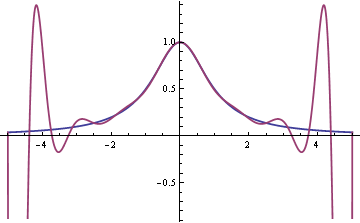The error near the ends is terrible, though the fit does improve in the middle. If instead of using evenly spaced nodes you use the roots of Chebyshev polynomials, the interpolating polynomials do in fact converge, and converge quickly. If the kth derivative of f has bounded variation, then the error in interpolating f at n points is O(nk).# How to digitize a graph

Suppose you have a graph of a function, but you don’t have an equation for it or the data that produced it. How can you reconstruction the function?

There are a lot of software packages to digitize images. For example, Web Plot Digitizer is one you can use online. Once you have digitized the graph at a few points, you can fit a spline to the points to approximately reconstruct the function. Then as a sanity check, plot your reconstruction to see if it looks like the original. It helps to have the same aspect ratio so you’re not distracted by something that doesn’t matter, and so that differences that do matter are easier to see.

For example, here is a graph from Zwicker and Fastl’s book on psychoacoustics. It contains many graphs with no data or formulas. This particular one gives the logarithmic transmission factor between free field and the peripheral hearing system.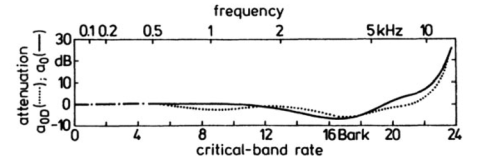Here’s Python code to reconstruct the functions behind these two curves.

import matplotlib.pyplot as plt
import numpy as np
from scipy import interpolate

curve_names = ["Free", "Diffuse"]
plot_styles = { "Free" : 'b-', "Diffuse" : 'g:'}

data = {}
for name in curve_names:

x = data[:,0]
y = data[:,1]
spline = interpolate.splrep(x, y)
xnew = np.linspace(0, max(x), 100)
ynew = interpolate.splev(xnew, spline, der=0)
plt.plot(xnew, ynew, plot_styles[name])

logical_x_range  = 24    # Bark
logical_y_range  = 40    # dB
physical_x_range = 7     # inch
physical_y_range = 1.625 # inch

plt.legend(curve_names, loc=2)
plt.xlabel("critical-band rate")
plt.ylabel("attenuation")
plt.xlim((0, logical_x_range))

plt.axes().set_aspect(
(physical_y_range/logical_y_range) /
(physical_x_range/logical_x_range) )
ax = plt.gca()
ax.get_xaxis().set_ticks([0, 4, 8, 12, 16, 20, 24])
ax.get_yaxis().set_ticks([-10, 0, 10, 20, 30])

plt.show()



Here’s the reconstructed graph.